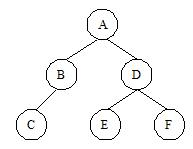bolt
Try our new interface for solving problems
Problems

# Pre, in and post

Time limit 1 second
Memory limit 128 MiB

A common problem in data structures is to determine the traversal of a binary tree. There are three classic ways to do it:

Pre-order: You must visit in sequence the root, left subtree and the right subtree.In-order: You must visit in sequence the left subtree, the root and the right subtree.Post-order: You must visit in sequence the left subtree, the right subtree and the root.

See the picture below:The pre, in and post-order traversal are, respectively, ABCDEF, CBAEDF and CBEFDA. In this problem, you must compute the post-order traversal of a binary tree given its in-order and pre-order traversals.

## Input data

The input set consists of a positive number c2000, that gives the number of test cases and c lines, one for each test case. Each test case starts with a number n (1n52), the number of nodes in this binary tree. After, there will be two strings S and S that describe the pre-order and in-order traversal of the tree. The nodes of the tree are labeled with different characters in the range a..z and A..Z. The values of n, S and S are separeted by a blank space.

## Output data

For each input set, you should output a line containing the post-order transversal for the current tree.

## Examples

Input example #1
3
3 xYz Yxz
3 abc cba
6 ABCDEF CBAEDF

Output example #1
Yzx
cba
CBEFDA